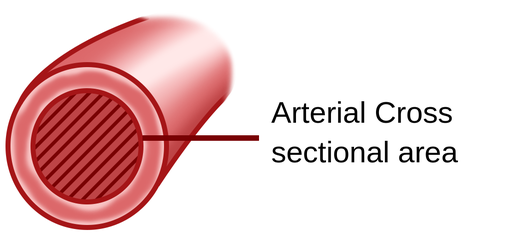The Doppler effect is the change in frequency or wavelength of a wave as the source and observer move towards, or away from, each other.

The most relatable case of Doppler effect is a car with a siren approaching a pedestrian. When the car is moving towards the pedestrian the pitch of the sound will be higher, meaning that there is an increase in the frequency of the wave, while once the car has passed the pedestrian and moving further away, the pitch turns lower, which means that there is a decrease in the frequency of the wave.

One of the many applications of the Doppler effect is to determine the speed of a moving object. If a wave is produced and it rebounds on a moving object, the shift in the frequency produced by the rebound can be used to determine the speed of the moving object.

The Doppler effect also has medical applications. The shift in the frequency can be used to determine the velocity of blood flow. The produced ultrasound wave can rebound on the many different cells within the blood and provide an accurate measurement of blood velocity in the veins and arteries. However, if a measurement of blood flow is required, for example, when calculating cardiac output, you must multiply the velocity times the cross-sectional area of the vessel to get the flow rate. The velocity is measured in cm/sec, the cross-sectional area of the vessel is measured in cm2 and the flow rate is measured in cm3/sec .Figure 1. Arterial cross-section.